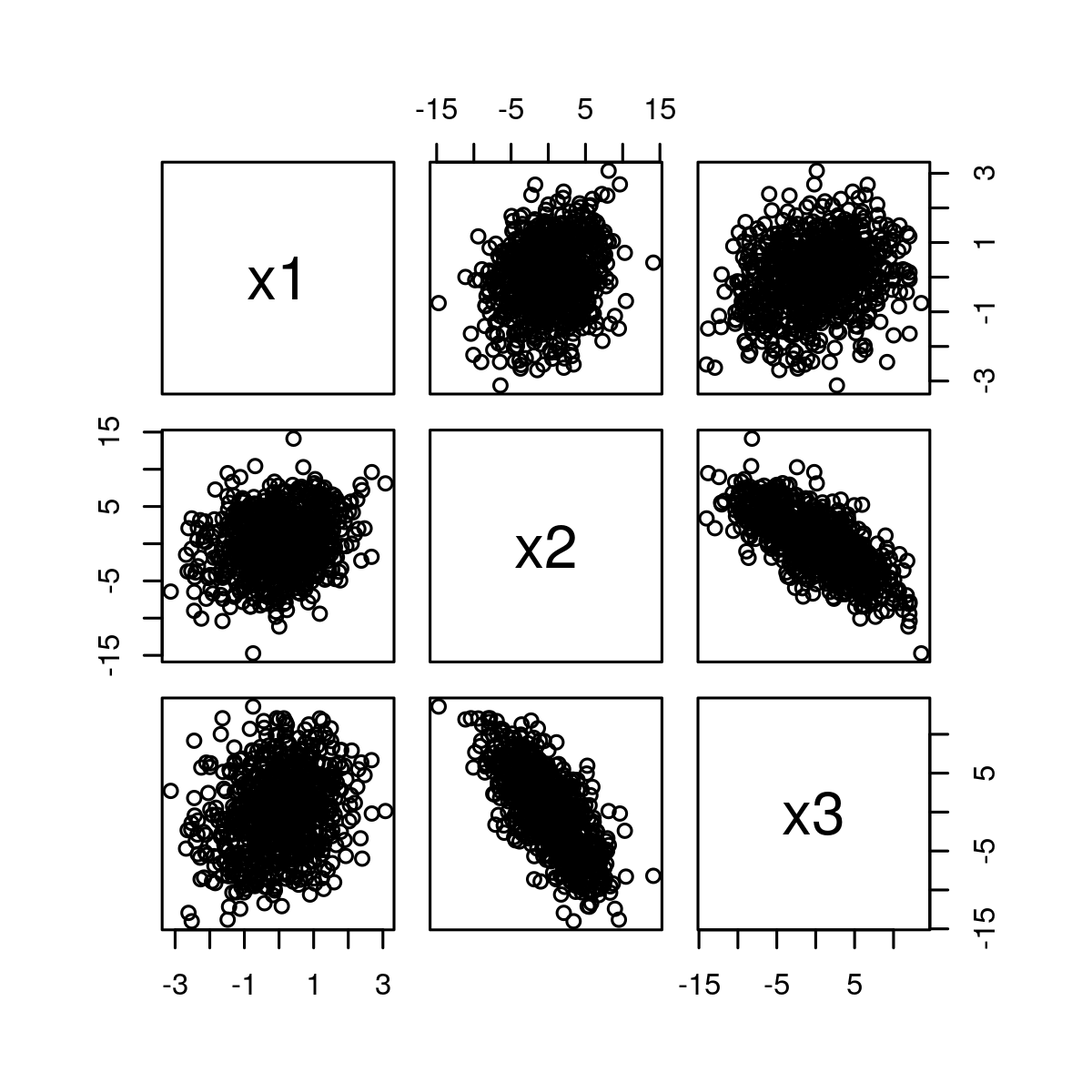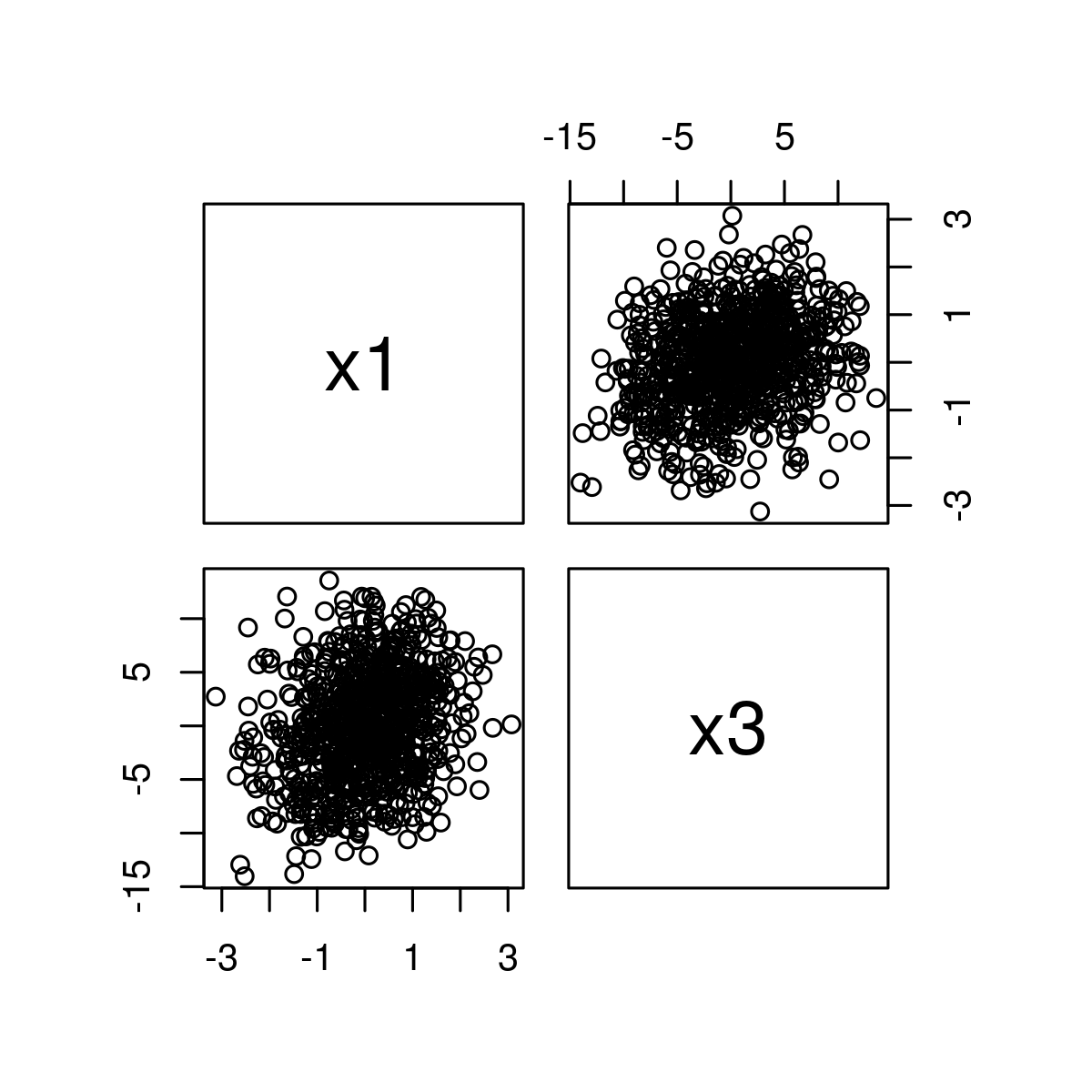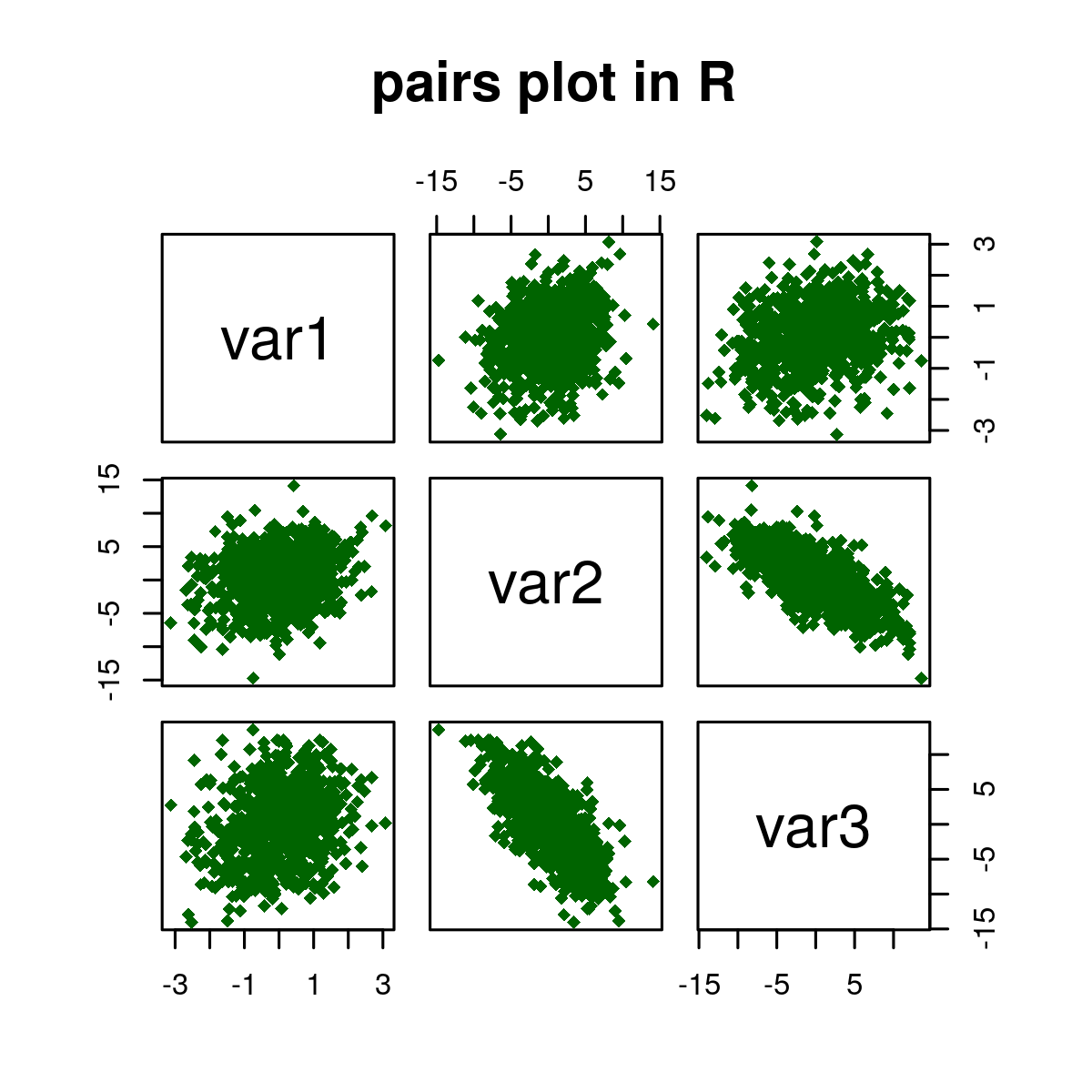# Create a Plot Matrix of Scatterplots in R Programming – pairs() Function

`pairs()` function in R language is used to return a plot matrix, consisting of scatter plots corresponding to each data frame.

Syntax: pairs(data)

Parameters:
data: It is defined as  value of pairs Plot.

Returns:
Color, Labels, Panels, and by Group in pairs plot.

Example 1: Basic example to illustrate pair()

 `# Set seed for reproducibility ` `set.seed``(425340) ` ` `  `# Sample size of 800 ` `N <- 800  ` `  `  `# Create variable ` `x1 <- ``rnorm``(N)  ` ` `  `# Create correlated variable ` `x2 <- x1 + ``rnorm``(N, 0, 4)   ` ` `  `# Create another correlated variable ` `x3 <- 2 * x1 - x2 + ``rnorm``(N, 0, 3)      ` `  `  `data <- ``data.frame``(x1, x2, x3)      ` `pairs``(data)                `

Output:Here, in the above example, the diagonal shows the names of the three numeric variables. The middle graphic in the first-row shows the relation between x1 and x2 whereas the right graph in the first row shows the relation between x1 and x3 and so on.

Example 2: Another example to select Variables of pairs Plot

 `# Set seed for reproducibility ` `set.seed``(425340) ` ` `  `# Sample size of 800 ` `N <- 800       ` `  `  `# Create variable ` `x1 <- ``rnorm``(N)  ` ` `  `# Create correlated variable ` `x2 <- x1 + ``rnorm``(N, 0, 4)              ` ` `  `# Create another correlated variable ` `x3 <- 2 * x1 - x2 + ``rnorm``(N, 0, 3)      ` `  `  `data <- ``data.frame``(x1, x2, x3)      ` ` `  `pairs``(~ x1 + x3, data = data)     `

Output:Example 3:Another example with implementation to Modify Color, Shape of Points, Labels and Title

 `# Set seed for reproducibility ` `set.seed``(425340) ` ` `  `# Sample size of 800 ` `N <- 800    ` ` `  `# Create variable ` `x1 <- ``rnorm``(N)    ` ` `  `# Create correlated variable ` `x2 <- x1 + ``rnorm``(N, 0, 4)    ` ` `  `# Create another correlated variable ` `x3 <- 2 * x1 - x2 + ``rnorm``(N, 0, 3)      ` `  `  `data <- ``data.frame``(x1, x2, x3)      ` ` `  `pairs``(~ x1 + x2 + x3, data = data) ` `pairs``(~ x1 + x3, data = data)     ` `pairs``(data[, 1:3], ` `      ``col = ``"darkgreen"``,    ``# Change color                       ` `      ``pch = 18,             ``# Change shape of points ` `       `  `      ``# Change labels of diagonal ` `      ``labels = ``c``(``"var1"``, ``"var2"``, ``"var3"``),    ` `      ``main = ``" pairs plot in R"``)    `

Output:My Personal Notes arrow_drop_upCheck out this Author's contributed articles.

If you like GeeksforGeeks and would like to contribute, you can also write an article using contribute.geeksforgeeks.org or mail your article to contribute@geeksforgeeks.org. See your article appearing on the GeeksforGeeks main page and help other Geeks.

Please Improve this article if you find anything incorrect by clicking on the "Improve Article" button below.

Article Tags :

Be the First to upvote.

Please write to us at contribute@geeksforgeeks.org to report any issue with the above content.